0
0
01. 云栖社区>
2. 博客>
3. 正文

随机数生成算法【详解，归纳】

angel_kitty 2017-05-31 22:27:00 浏览871

1、蒙特卡洛方法

a.针对实际问题建立一个简单且便于实现的概率统计模型，使所求的量恰好是该模型的概率分布或数字特征。
b.对模型的随机变量建立抽样方法，在计算机上进行模拟测试，抽取足够多的随机数。
c.对模拟实验结果进行统计分析，给出所求解的“估计”。
d.必要时，改进模型以提高估计精度和减少实验费用，提高模拟效率。

2、冯·诺依曼

3、U(0,1)随机数的产生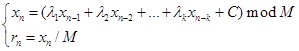4、从U(0,1)到其它概率分布的随机数

 X 0 1 2 P(x) 0.3 0.3 0.4

U是(0,1)的均匀分布，则有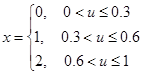，这样得到的x便具有X的分布律。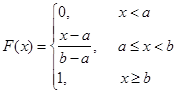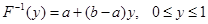。所以生成U(0,1)的随机数U，则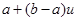便是来自U(a,b)的随机数。

1.已知随机分布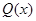且x的取值区间也为[a,b]，并要求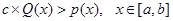，如图：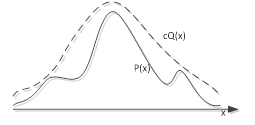2.从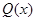中随机抽样得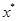，然后由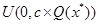的均匀分布抽样得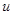3.接受或舍弃取样值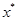，如果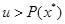舍弃该值；返回上一步，否则接受。几何解释如下：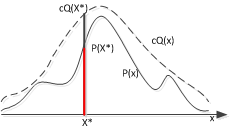5、正态随机数的生成Box Muller方法，设（X,Y）是一对相互独立的服从正态分布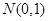的随机变量，则有概率密度函数：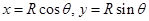，其中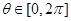，则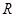有分布函数：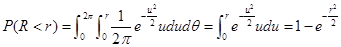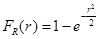，则分布函数的反函数得：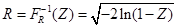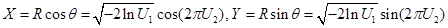X和Y均服从正态分布。用Box Muller方法来生成服从正态分布的随机数是十分快捷方便的。

1 生成随机数

1 #include <stdio.h>
2 #include <stdlib.h>
3 #include <time.h>
4
5 int main()
6 {
7     int i,j;
8     srand((int)time(0));
9     for (int i = 0; i < 10; i++)
10     {
11         for (int j = 0; j < 10; j++)
12         {
13             printf("%d  ",rand());
14         }
15         printf("\n");
16     }
17     return 0;
18 }

1 #include <stdio.h>
2 #include <stdlib.h>
3 #include <time.h>
4
5 int main()
6 {
7     int i,j;
8     srand((int)time(0));
9     for (int i = 0; i < 10; i++)
10     {
11         for (int j = 0; j < 10; j++)
12         {
13             printf("%d  ",rand()*100/32767);
14         }
15         printf("\n");
16     }
17     return 0;
18 }

1 #include <stdio.h>
2 #include <stdlib.h>
3 #include <time.h>
4
5 int main()
6 {
7     int i,j;
8     srand((int)time(0));
9     for (int i = 0; i < 10; i++)
10     {
11         for (int j = 0; j < 10; j++)
12         {
13             printf("%d  ",rand()/1000+100);
14         }
15         printf("\n");
16     }
17     return 0;
18 }

1 #include<iostream>
2 #include<ctime>
3 using namespace std;
4 int main()
5 {
6  srand(time(0));
7  for(int i=0;i<10;i++)
8  {
9   //产生10以内的整数
10   cout<<rand()%10<<endl;
11  }
12 }
2 生成[0,1]之间均匀分布的随机数算法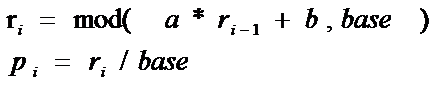（1）这里的取模运算是针对浮点型数据的，而c语言中的取模运算不能用于浮点数数据的操作，这样就需要用户自己编写取模的程序；
（2）ri是随着递推而每次更新的。因此，如果将这个算法编写出函数，需要考虑参数是传值还是传地址；

1 #include <stdio.h>
2
3
4 double rand0_1(double *r)
5 {
6     double base=256.0;
7     double a=17.0;
8     double b=139.0;
9     double temp1=a*(*r)+b;
10     //printf("%lf",temp1);
11     double temp2=(int)(temp1/base); //得到余数
12     double temp3=temp1-temp2*base;
13     //printf("%lf\n",temp2);
14     //printf("%lf\n",temp3);
15     *r=temp3;
16     double p=*r/base;
17     return p;
18 }
19
20 int main()
21 {
22     double r=5.0;
23     printf("output 10 number between 0 and 1:\n");
24     for (int i = 0; i < 10; i++)
25     {
26         printf("%10.5lf\n",rand0_1(&r));
27     }
28     return 0;
29 }
3 产生任意范围内的随机数，比如产生[m,n]之间的随机数

m+(m-n)*rand0_1(&r)就行了；
1 #include <stdio.h>
2
3
4 double rand0_1(double *r)
5 {
6     double base=256.0;
7     double a=17.0;
8     double b=139.0;
9     double temp1=a*(*r)+b;
10     //printf("%lf",temp1);
11     double temp2=(int)(temp1/base); //得到余数
12     double temp3=temp1-temp2*base;
13     //printf("%lf\n",temp2);
14     //printf("%lf\n",temp3);
15     *r=temp3;
16     double p=*r/base;
17     return p;
18 }
19
20 int main()
21 {
22     double m=1.0,n=5.0;
23     double r=5.0;
24     printf("output 10 number between 0 and 1:\n");
25     for (int i = 0; i < 10; i++)
26     {
27         printf("%10.5lf\n",m+(n-m)*rand0_1(&r));
28     }
29     return 0;
30 }
4 正态分布的随机数生成算法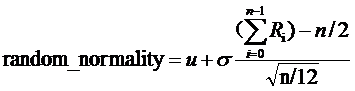u为均值，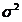为方差，当n趋向于无穷大的时候，得到随机的随机分布为正态分布；

1 #include <stdio.h>
2 #include <math.h>
3
4 double rand0_1(double *r)
5 {
6       double base=256.0;
7       double a=17.0;
8       double b=139.0;
9       double temp1=a*(*r)+b;
10       //printf("%lf",temp1);
11       double temp2=(int)(temp1/base); //得到余数
12       double temp3=temp1-temp2*base;
13       //printf("%lf\n",temp2);
14       //printf("%lf\n",temp3);
15       *r=temp3;
16       double p=*r/base;
17       return p;
18 }
19
20 double random_normality(double u,double t,double *r ,double n)
21 {
22       double total=0.0;
23       double result;
24       for (int i = 0; i < n; i++)
25       {
26             total+=rand0_1(r);
27       }
28       result=u+t*(total-n/2)/sqrt(n/12);
29       return result;
30 }
31
32 int main()
33 {
34       double r=5.0;
35       double u=2.0;
36       double t=3.5;
37       double n=12;
38       printf("output 10 number between 0 and 1:\n");
39       for (int i = 0; i < 10; i++)
40       {
41             printf("%10.5lf\n",random_normality(u,t,&r,n));
42       }
43       return 0;
44 }

补充知识点：leveldb中使用了一个简单的方式来实现随机化数；算法的核心是seed_ = (seed_ * A) % M,

1 private:
2   uint32_t seed_;
3  public:
4   explicit Random(uint32_t s) : seed_(s & 0x7fffffffu) {
5     // Avoid bad seeds.
6     if (seed_ == 0 || seed_ == 2147483647L) {
7       seed_ = 1;
8     }
9   }
10   uint32_t Next() {
11     static const uint32_t M = 2147483647L;   // 2^31-1
12     static const uint64_t A = 16807;  // bits 14, 8, 7, 5, 2, 1, 0
13     // We are computing
14     //       seed_ = (seed_ * A) % M,    where M = 2^31-1
15     //
16     // seed_ must not be zero or M, or else all subsequent computed values
17     // will be zero or M respectively.  For all other values, seed_ will end
18     // up cycling through every number in [1,M-1]
19     uint64_t product = seed_ * A;
20
21     // Compute (product % M) using the fact that ((x << 31) % M) == x.
22     seed_ = static_cast<uint32_t>((product >> 31) + (product & M));
23     // The first reduction may overflow by 1 bit, so we may need to
24     // repeat.  mod == M is not possible; using > allows the faster
25     // sign-bit-based test.
26     if (seed_ > M) {
27       seed_ -= M;
28     }
29     return seed_;
30   }
31   // Returns a uniformly distributed value in the range [0..n-1]
32   // REQUIRES: n > 0
33   uint32_t Uniform(int n) { return Next() % n; }
34
35   // Randomly returns true ~"1/n" of the time, and false otherwise.
36   // REQUIRES: n > 0
37   bool OneIn(int n) { return (Next() % n) == 0; }
38
39   // Skewed: pick "base" uniformly from range [0,max_log] and then
40   // return "base" random bits.  The effect is to pick a number in the
41   // range [0,2^max_log-1] with exponential bias towards smaller numbers.
42   uint32_t Skewed(int max_log) {
43     return Uniform(1 << Uniform(max_log + 1));
44   }
45 };

C语言/C++怎样产生随机数：这里要用到的是rand()函数, srand()函数,和time()函数。

srand()函数定义 ： void srand (unsigned int seed);

time(0)或者time(NULL)返回的是系统的时间（从1970.1.1午夜算起），单位：秒

angel_kitty
+ 关注